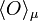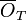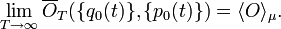Ergodic hypothesis

The Ergodic hypothesis essentially states that an ensemble average (i.e. an instance of a Monte Carlo simulation) of an observable,$\langle O \rangle_\mu$ is equivalent to the time average,$\overline{O}_T$ of an observable (i.e. molecular dynamics). i.e.$\lim_{T \rightarrow \infty} \overline{O}_T (\{q_0(t)\},\{p_0(t)\}) = \langle O \rangle_\mu.$